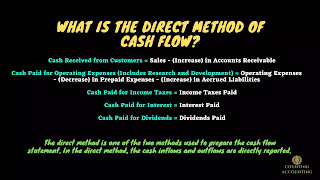# Direct Method of Cash flow

The cash flow statement is a financial statement that summarizes the cash inflows and outflows of a company during a specific period. The cash flow statement is one of the most important financial statements as it helps investors and stakeholders understand the liquidity of the company. In this article, we will discuss the direct method of preparing the cash flow statement, including the formulas used to calculate the different components of the cash flow statement.

##What is the Direct Method of Cash flow?

The direct method is one of the two methods used to prepare the cash flow statement. In the direct method, the cash inflows and outflows are directly reported. This means that the direct method reports the actual cash received and paid during the period, rather than using accrual accounting to estimate the cash flows.

## Formulas of the Direct Method of Cash flow

The direct method uses the following formulas to calculate the different components of the cash flow statement:

Cash Received from Customers = Sales - (Increase) in Accounts Receivable

Cash Paid for Operating Expenses (Includes Research and Development) = Operating Expenses - (Decrease) in Prepaid Expenses - (Increase) in Accrued Liabilities

Cash Paid for Income Taxes = Income Taxes Paid

Cash Paid for Interest = Interest Paid

Cash Paid for Dividends = Dividends Paid

## Explanation of the Formulas

The formula for cash received from customers is calculated by subtracting the increase in accounts receivable from the total sales revenue. Accounts receivable represents the money that the company is owed by its customers, and an increase in accounts receivable means that the company has not yet received payment for sales made during the period. By subtracting the increase in accounts receivable from the total sales revenue, we can calculate the actual cash received from customers during the period.

Cash Paid for Operating Expenses

The formula for cash paid for operating expenses is calculated by subtracting the decrease in prepaid expenses and adding the increase in accrued liabilities from the total operating expenses. Prepaid expenses represent expenses that have been paid in advance, and a decrease in prepaid expenses means that the company has used some of the prepaid expenses during the period. Accrued liabilities represent expenses that the company has incurred but has not yet paid, and an increase in accrued liabilities means that the company has incurred more expenses during the period. By subtracting the decrease in prepaid expenses and adding the increase in accrued liabilities from the total operating expenses, we can calculate the actual cash paid for operating expenses during the period.

Cash Paid for Income Taxes, Interest, and Dividends

The formulas for cash paid for income taxes, interest, and dividends are straightforward. These components represent the actual cash payments made by the company during the period for income taxes, interest, and dividends.

## Example of Cash flow (Direct Method)

ABC Company
Cash Flow Statement (Direct Method)
For the Year Ended December 31, 2022
Cash Flow from Operating Activities
Cash Receipts
From Customers \$500,000
Cash Payments
For Operating Expenses (\$300,000)
For Income Tax (\$50,000)
For Interest (\$10,000)
For Dividends (\$20,000)
Net Cash Flow from Operating Activities \$120,000
Cash Flow from Investing Activities
Cash Flow from Financing Activities
Net Increase in Cash and Cash Equivalents \$120,000
Beginning Cash and Cash Equivalents \$50,000
Ending Cash and Cash Equivalents \$170,000

In this example, we have calculated the cash flows for ABC Company using the direct method. The cash inflows from customers during the year were \$500,000, while the cash outflows for operating expenses, income tax, interest, and dividends were \$300,000, \$50,000, \$10,000, and \$20,000, respectively. The net cash flow from operating activities was \$120,000.

The cash flow statement also includes sections for cash flow from investing activities and cash flow from financing activities, but they have been left blank in this example as we do not have any relevant data for them. Finally, we calculate the net increase in cash and cash equivalents for the year, which was \$120,000, and the ending balance of cash and cash equivalents was \$170,000.

## Conclusion

The direct method of preparing the cash flow statement is a straightforward approach that reports the actual cash inflows and outflows of the company during the period. By using the formulas discussed in this article, we can calculate the different components of the cash flow statement and understand the liquidity of the company. The direct method provides valuable information to investors and stakeholders and should be considered when preparing the cash flow statement.